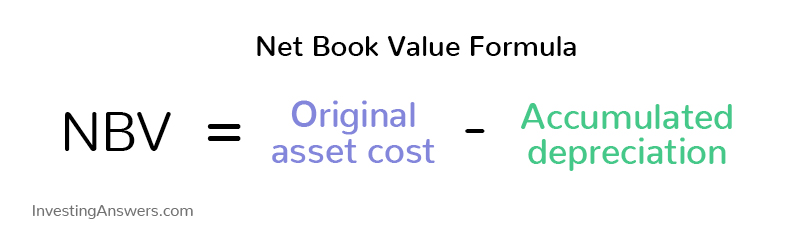# Net Book Value

Updated June 22, 2021

## What Is Net Book Value?

Net book value is the net value of an asset carried on its balance sheet.

Net book value results from the accounting technique of depreciating or amortizing the value of an asset: a company gradually “uses up” or expenses the cost of a fixed asset over the asset’s useful life. It’s one of several ways to derive a valuation for the asset but it may not equal the market price of the fixed asset.

## Why Is Net Book Value Important?

Net book value is an important metric to indicate a minimum/floor value of a company’s assets. This valuation is important when the company could be sold or liquidated. It helps a company keep more conservative  accounting records when estimating its total value.

### Where Is Net Book Value on the Balance Sheet?

Net book value is reported for the long-term (fixed) assets on the balance sheet.

## Net Book Value Formula

NBV can be calculated by taking a company’s original asset value and subtracting its total depreciation or amortization expense to date.The following is the NBV formula:Where:

• Original asset cost =  Any acquisition costs associated with the asset (this can include its purchase price delivery and handling costs, setup fees, and customs duties).

• Accumulated depreciation = depreciation (or amortization) expense per year * number of years

## How to Calculate Net Book Value

Let’s say ABC Trucking Company purchases a semi truck for \$100,000 and it has depreciated \$7,000 each year for five years.

Here’s how to derive NBV using the above net book value formula:

NBV = \$100,000 - (\$7,000 x 5 years) = \$65,000

This means the net book value of the truck would be \$65,000 after five years.

### Subtracting Depreciation, Amortization or Depletion

When learning how to calculate net book value, it’s important to understand how depreciation, amortization, or depletion plays a role in NBV.

Every asset has a period of time when it’s considered useful. For instance, a piece of machinery will have a certain life span until it’s no longer worth it to keep in a company’s regular roster. Since assets will lose some value over time, calculations need to consider the net book value depreciation (or amortization) of the asset’s value in relation to the asset’s original cost.

An asset’s NBV should decrease predictably and steadily until the end of its useful life. At that time, the NBV should be the equivalent of the asset’s salvage value, the value that it can be sold for at the end of its useful life.

## Net Book Value vs. Fair Market Value

Although both net book and fair market values are useful measures, an asset’s net book value may be different from its fair market value. Net book value is based on depreciation, which is an estimate based on the asset’s estimated useful life and salvage value. Net book value of machinery or equipment can also be impaired by damage or obsolescence. Fair market value should reflect the current price of the asset, based on its condition, which is often estimated as the replacement cost of the asset.

All of our content is verified for accuracy by Rachel Siegel, CFA and our team of certified financial experts. We pride ourselves on quality, research, and transparency, and we value your feedback. Below you'll find answers to some of the most common reader questions about Net Book Value.

### What Is the Net Book Value Formula for Depreciation?

Net book value formula for depreciation is an important part of calculating NBV because assets will have a certain period of time where they’re considered useful. Depreciation expense is calculated by applying this formula to estimate the amount of value used up in each period of the asset’s life. This needs to be taken into consideration as NBV will decrease over time until the end of an asset’s useful life.

### Is Net Book Value the Same as Book Value of Equity?

Book value can refer to the company’s equity value, specifically in relation to its stock value. It’s calculated by subtracting the company’s liabilities or debts from its assets. This figure is most often used in comparison to a company’s market capitalization (or stock value).

Rachel Siegel, CFA - 141Rachel Siegel, CFACFA Charterholder

Chartered Financial Analyst

Rachel Siegel, CFA is one of the nation's leading experts at ensuring the accuracy of financial and economic text.  Her prestigious background includes over 10 years creating professional financial certification exams and another 20 years of college-level teaching.